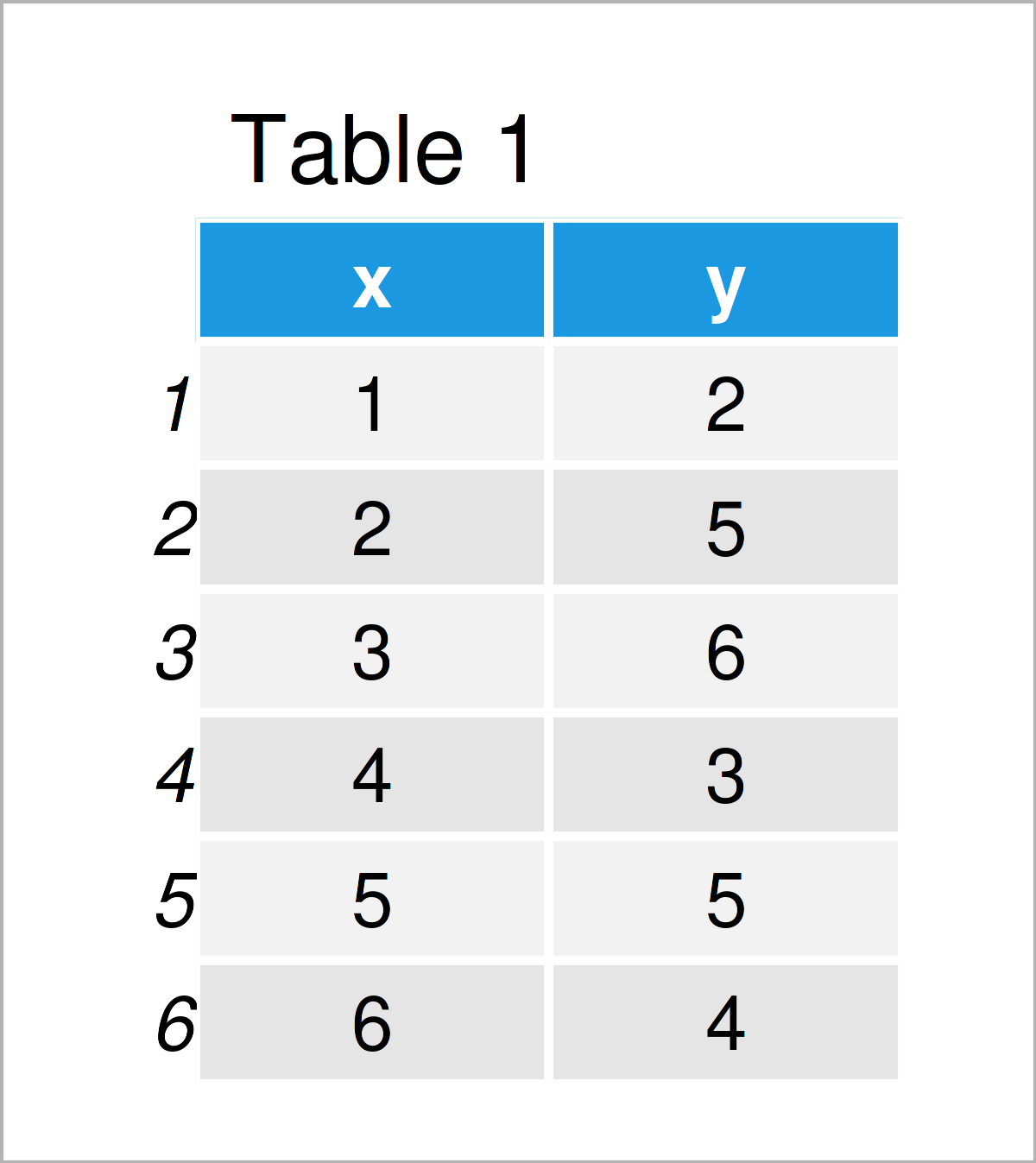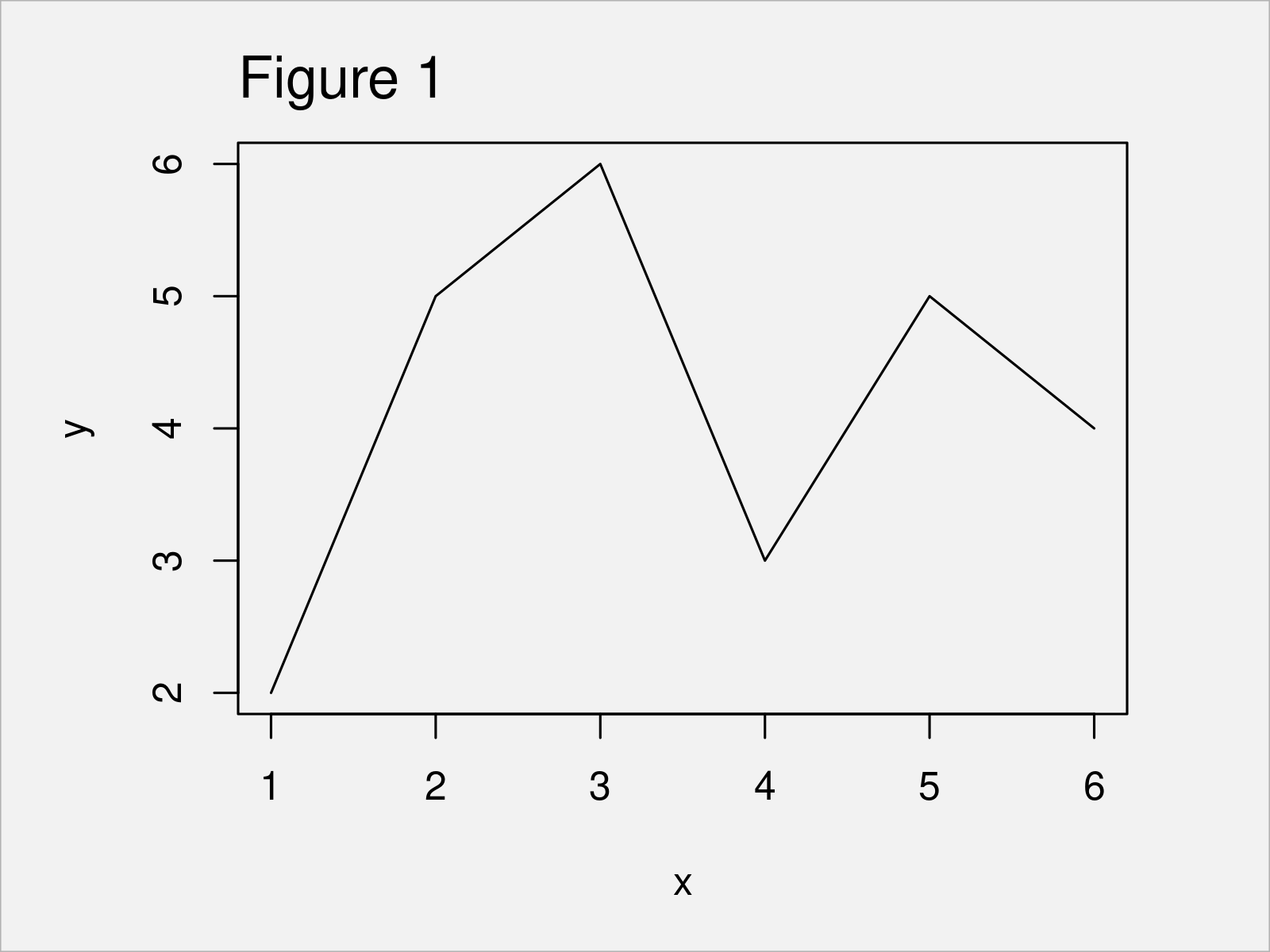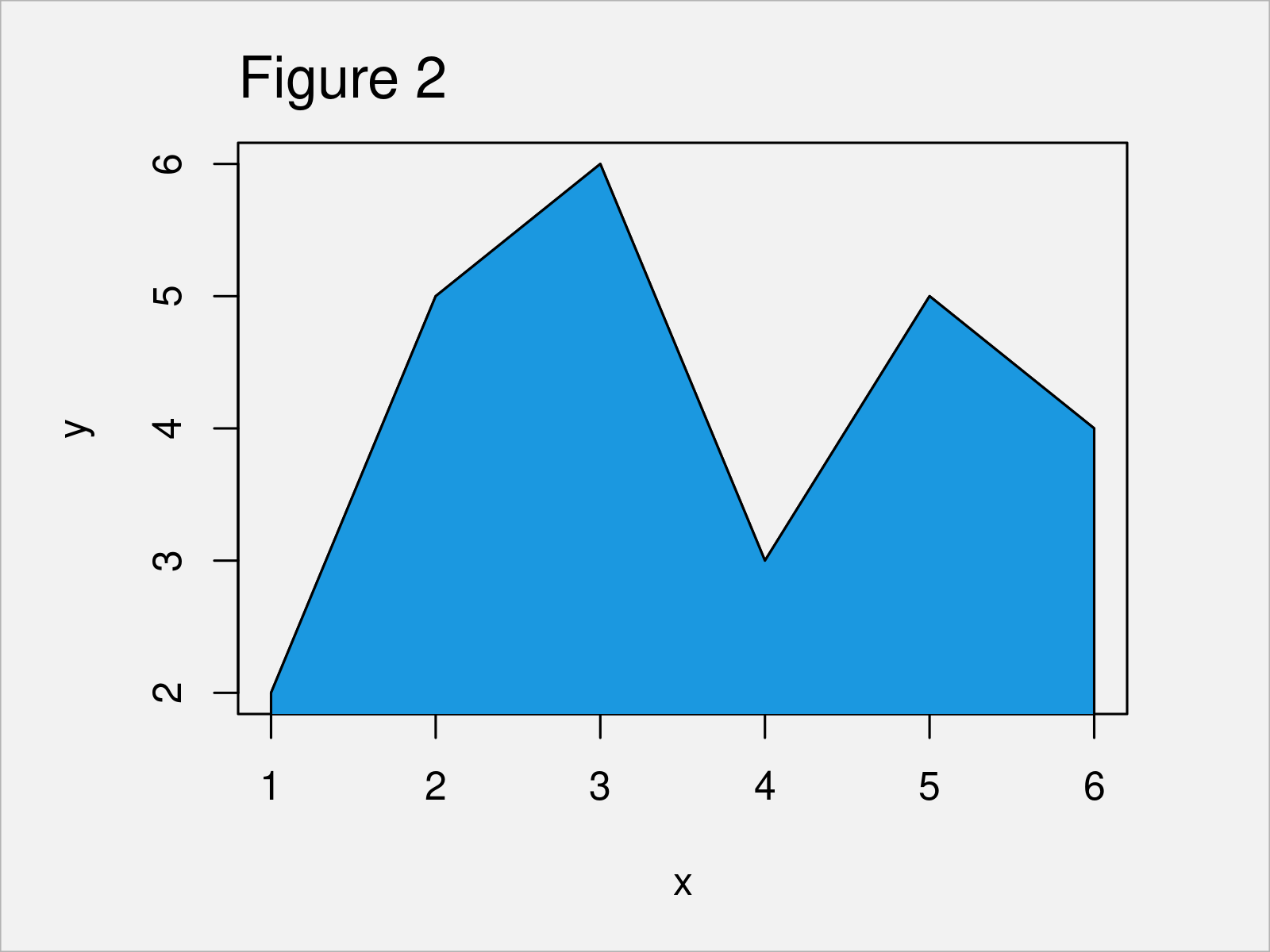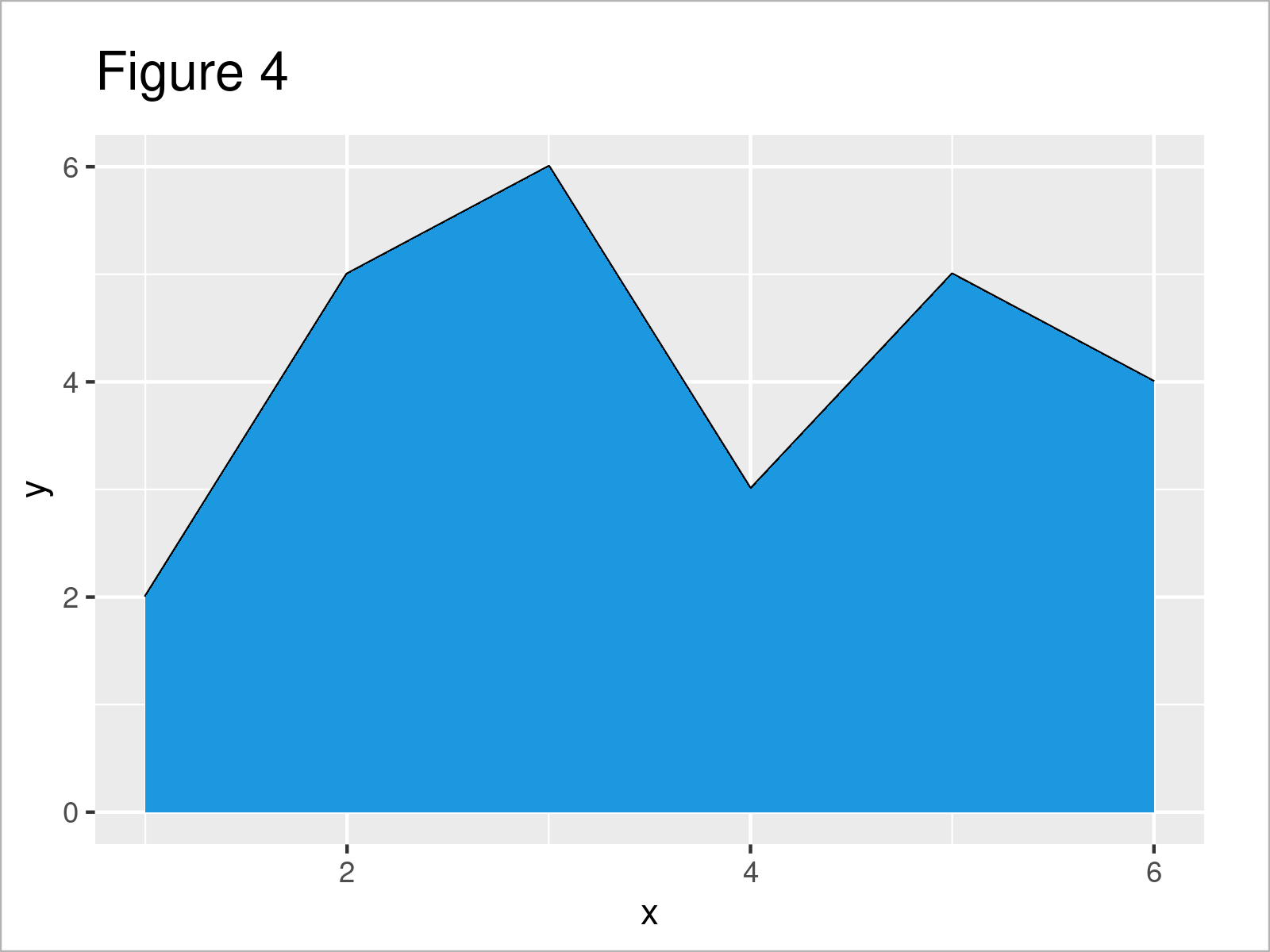# Fill Area Under Line Plot in R (2 Examples)

In this post you’ll learn how to add a filling color below the line in a line graph in R programming.

The tutorial looks as follows:

Let’s jump right to the R syntax!

## Creating Example Data

Consider the following example data:

```data <- data.frame(x = 1:6,         # Create example data frame
y = c(2, 5, 6, 3, 5, 4))
data                                # Print example data frame```As you can see based on Table 1, our exemplifying data is a data frame consisting of six rows and two variables.

## Example 1: Fill Area Under Line Plot Using Base R

Example 1 illustrates how to draw a Base R line graph with a filling color below the line.

Let’s first create a line plot without filling color:

`plot(data, type = "l")              # Draw Base R line plot without filled area`By running the previous R code, we have created Figure 1, i.e. a Base R line graphic without filling color.

Let’s add some color to the area under the line!

To accomplish this, we can use the polygon function as shown below:

```plot(data, type = "l")              # Fill area below line
polygon(x = c(min(data\$x), data\$x, max(data\$x)),
y = c(0, data\$y, 0),
col = "#1b98e0")```As shown in Figure 2, we have created a Base R line plot with color under the line curve.

## Example 2: Fill Area Under Line Plot Using ggplot2 Package

In Example 2, I’ll illustrate how to add color below the line of a ggplot2 graphic.

First, we have to install and load the ggplot2 package:

```install.packages("ggplot2")         # Install & load ggplot2
library("ggplot2")```

Next, let’s draw a ggplot2 plot without colors:

```ggp <- ggplot(data, aes(x, y)) +    # Draw ggplot2 line plot without filled area
geom_line()
ggp```Figure 3 illustrates the output of the previous code – A ggplot2 plot without filling color.

Now, we can add color to the graph using the geom_area function:

```ggp +                               # Fill area below line
geom_area(fill = "#1b98e0")```As shown in Figure 4, the previous R code has added some color below the line.

Note: The ggplot2 package changes the y-axis to start at zero when using the geom_area function. This is a difference compared to Base R.

In case you want to keep the y-axis limits, you may use the geom_ribbon function instead:

```ggp +                               # Filled area with same y-axis limits
geom_ribbon(aes(ymin = min(y),
ymax = y),
fill = "#1b98e0")```## Video & Further Resources

Have a look at the following video on my YouTube channel. In the video, I’m explaining the R programming code of this article in RStudio.

Please accept YouTube cookies to play this video. By accepting you will be accessing content from YouTube, a service provided by an external third party.If you accept this notice, your choice will be saved and the page will refresh.

In addition, you might want to read the related tutorials on my website. I have released numerous tutorials on topics such as dates, plot legends, lines, and graphics in R:

In this post you have learned how to change the filling color below the line in a line plot in the R programming language. In case you have further comments or questions, let me know in the comments section below.

Subscribe to the Statistics Globe Newsletter

•Ana Martins
July 5, 2023 5:20 pm

Is it possible to fill an area with two colours (that is, below zero in blue for example) and above zero in red (on the same graphic)?

•July 7, 2023 7:45 am